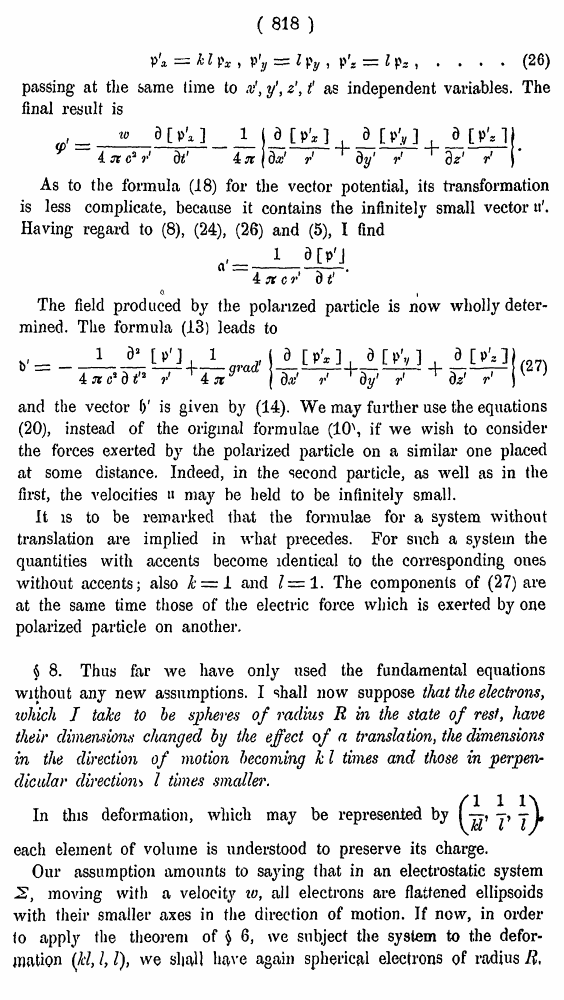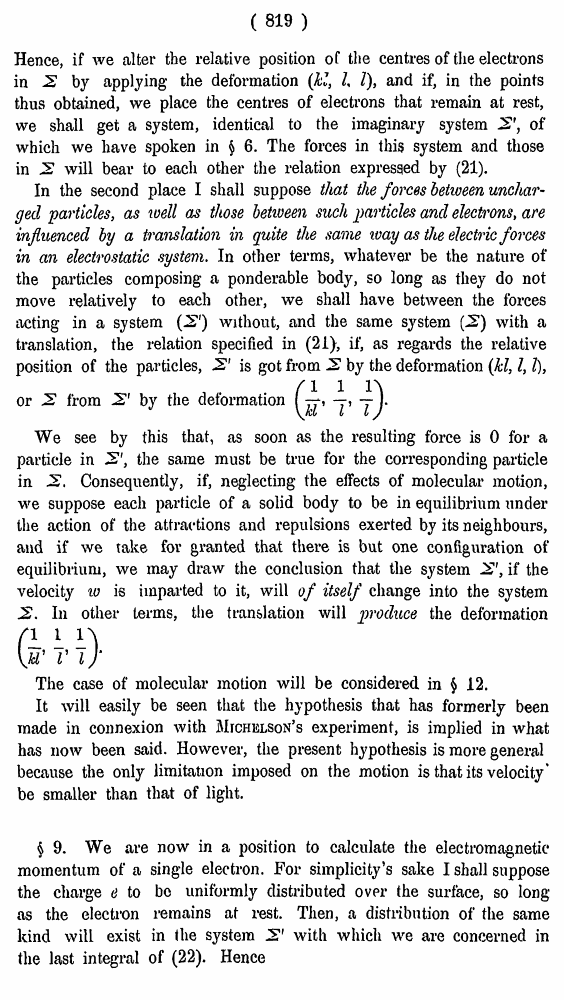# H. A. Lorentz. Electromagnetic phenomena in a system moving with any velocity smaller than that of light. // Proceedings Royal Acad., Amsterdam. Vol. VI., 1904

В начало   Другие форматы   <<<     Страница 818   >>>809  810  811  812  813  814  815  816  817  818 819  820  821  822  823  824  825  826  827  828  829  830  831 ( 818 ) .... (26) passing at the same time to x', y\ z', t as independent variables. The final result is As to the formula (18) for the vector potential, its transformation is less complicate, because it contains the infinitely small vector Having regard to (8), (24), (26) and (5), I find « i The field produced by the polarized particle is now wholly determined. The formula (13) leads to (27) and the vector f)' is given by (14). We may further use the equations (20), instead of the original formulae (10\ if we wish to consider the forces exerted by the polarized particle on a similar one placed at some distance. Indeed, in the second particle, as well as in the first, the velocities » may be held to be infinitely small. It is to be remarked that the formulae for a system without translation are implied in what precedes. For such a system the quantities with accents become identical to the corresponding ones without accents; also k — 1 and I— 1. The components of (27) are at the same time those of the electric force which is exerted by one polarized particle on another. § 8. Thus far we have only used the fundamental equations without any new assumptions. I shall now suppose that the electrons, which I take to be spheres of radius R in the state of rest, have their dimensions changed by the effect of a translation, the dimensions in the direction of motion becoming k I times and those in perpendicular direction•> I times smaller. In this deformation, which may be represented by each element of volume is understood to preserve its charge. Our assumption amounts to saying that in an electrostatic system 2, moving with a velocity to, all electrons are flattened ellipsoids with their smaller axes in the direction of motion. If now, in order (o apply the theorem of § 6, we subject the system to the deformation (Jcl, I, I), we sliaU have again spherical electrons of radius B, ( 819 ) Hence, if we alter the relative position of the centres of the electrons in 2 by applying the deformation (kl, I, I), and if, in the points thus obtained, we place the centres of electrons that remain at rest, we shall get a system, identical to the imaginary system 2', of which we have spoken in § 6. The forces in this system and those in 2 will bear to each other the relation expressed by (21). In the second place I shall suppose that the forces between uncharged particles, as well as those between such particles and electrons, are influenced by a translation in quite the same ivay as the electric forces in an electrostatic system. In other terms, whatever be the nature of the particles composing a ponderable body, so long as they do not move relatively to each other, we shall have between the forces acting in a system (2") without, and the same system (2) with a translation, the relation specified in (21), if, as regards the relative position of the particles, 2' is got from 2 by the deformation (kl, I, I), We see by this that, as soon as the resulting force is 0 for a particle in 2', the same must be true for the corresponding particle in 2, Consequently, if, neglecting the effects of molecular motion, we suppose each particle of a solid body to be in equilibrium under the action of the attractions and repulsions exerted by its neighbours, and if we take for granted that there is but one configuration of equilibrium, we may draw the conclusion that the system 2', if the velocity to is imparted to it, will of itself change into the system 2. In other terms, the translation will produce the deformation The case of molecular motion will be considered in § 12. It will easily be seen that the hypothesis that has formerly been made in connexion with Michelson's experiment, is implied in what has now been said. However, the present hypothesis is more general because the only limitation imposed on the motion is that its velocity* be smaller than that of light. \$ 9. We are now in a position to calculate the electromagnetic momentum of a single electron. For simplicity’s sake I shall suppose the charge e to be uniformly distributed over the surface, so long as the electron remains at rest. Then, a distribution of the same kind will exist in the system 2' with which we are concerned in the last integral of (22). Hence or 2 from 2' by the deformation